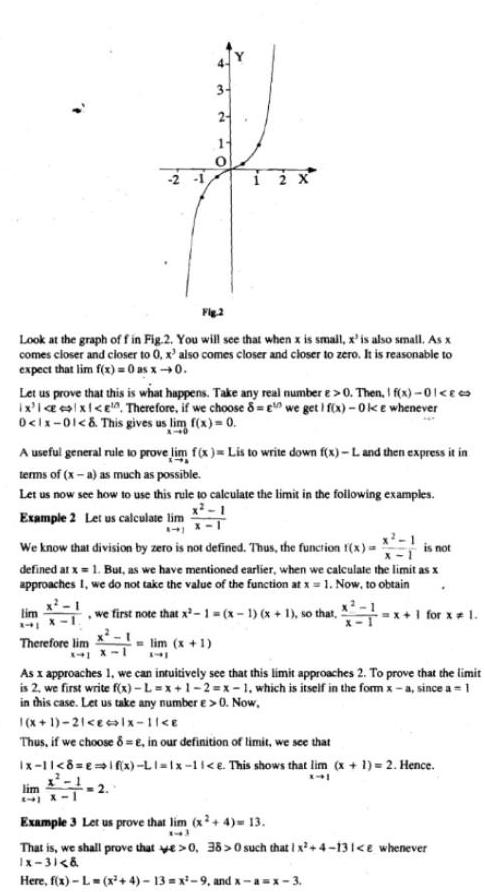Question:

# Fig 2 Look at the graph of f in Fig 2 You will see that when

Last updated: 9/17/2023Fig 2 Look at the graph of f in Fig 2 You will see that when x is small x is also small As x comes closer and closer to er to 0 x also comes closer and closer to zero It is reasonable to expect that lim f x 0 as x 0 Let us prove that this is what happens Take any real number e 0 Then I f x 01 ix i x e Therefore if we choose 8 we get 1 f x Oke whenever 0 1x 01 This gives us lim f x 0 3 2 A useful general rule to prove lim f x Lis to write down f x L and then express it in terms of x a as much as possible Let us now see how to use this rule to calculate the limit in the following examples x 1 Example 2 Let us calculate lim lim 241 12 X is not We know that division by zero is not defined Thus the function f x defined at x 1 But as we have mentioned earlier when we calculate the limit as x approaches I we do not take the value of the function at x 1 Now to obtain x 1 we first note that x 1 x 1 x 1 so that x 1 for x 1 lim x 1 741 Therefore lim As x approaches 1 we can intuitively see that this limit approaches 2 To prove that the limit is 2 we first write f x L x 1 2 x 1 which is itself in the form x a since a 1 in this case Let us take any number e 0 Now 1 x 1 21 E lx 1 E Thus if we choose 8 E in our definition of limit we see that x 1 x 1 8 E if x L1 1x 11 e This shows that lim x 1 2 Hence X41 1 1 X 1 Example 3 Let us prove that lim x 4 13 13 That is we shall prove that e 0 38 0 such that 1 x 4 131 e whenever Ix 3 Here f x L x 4 13 x 9 and x a x 3